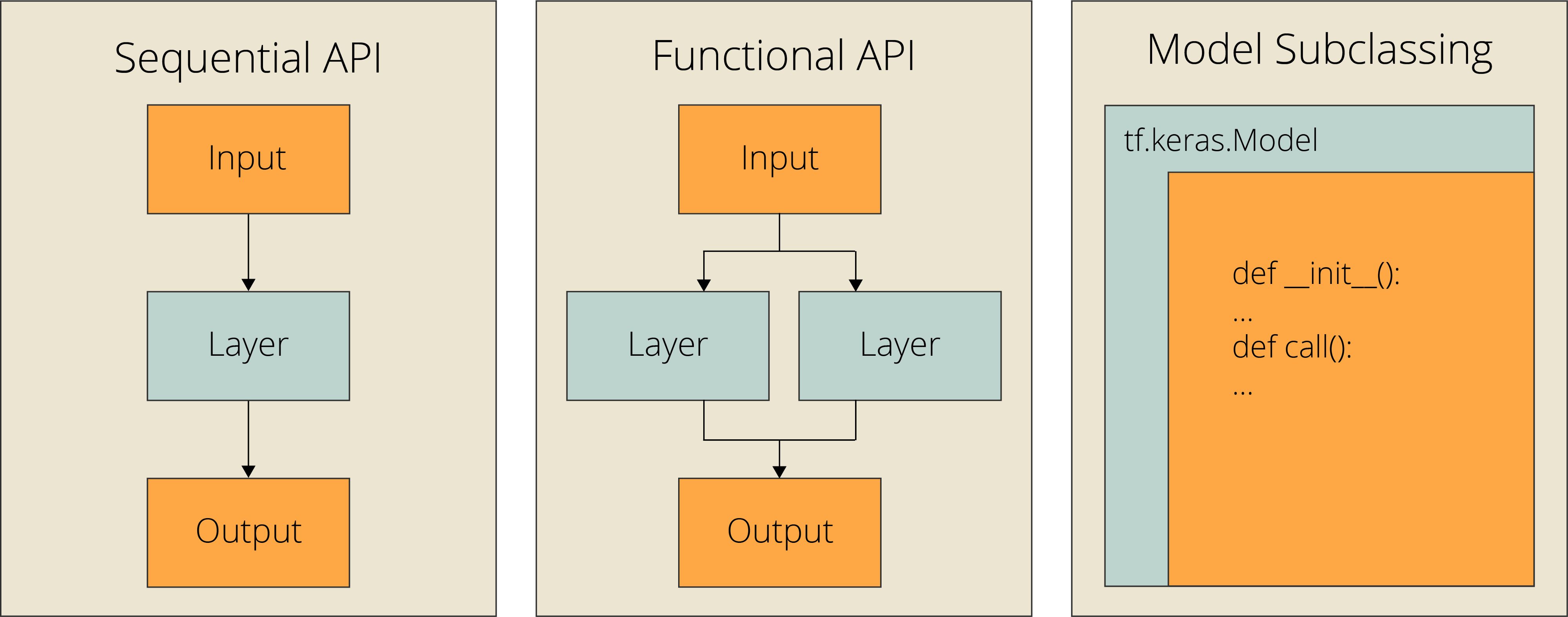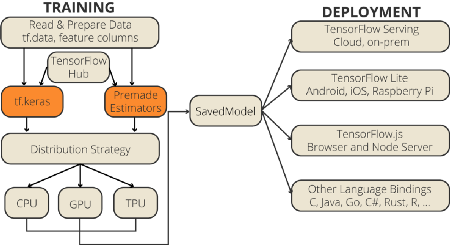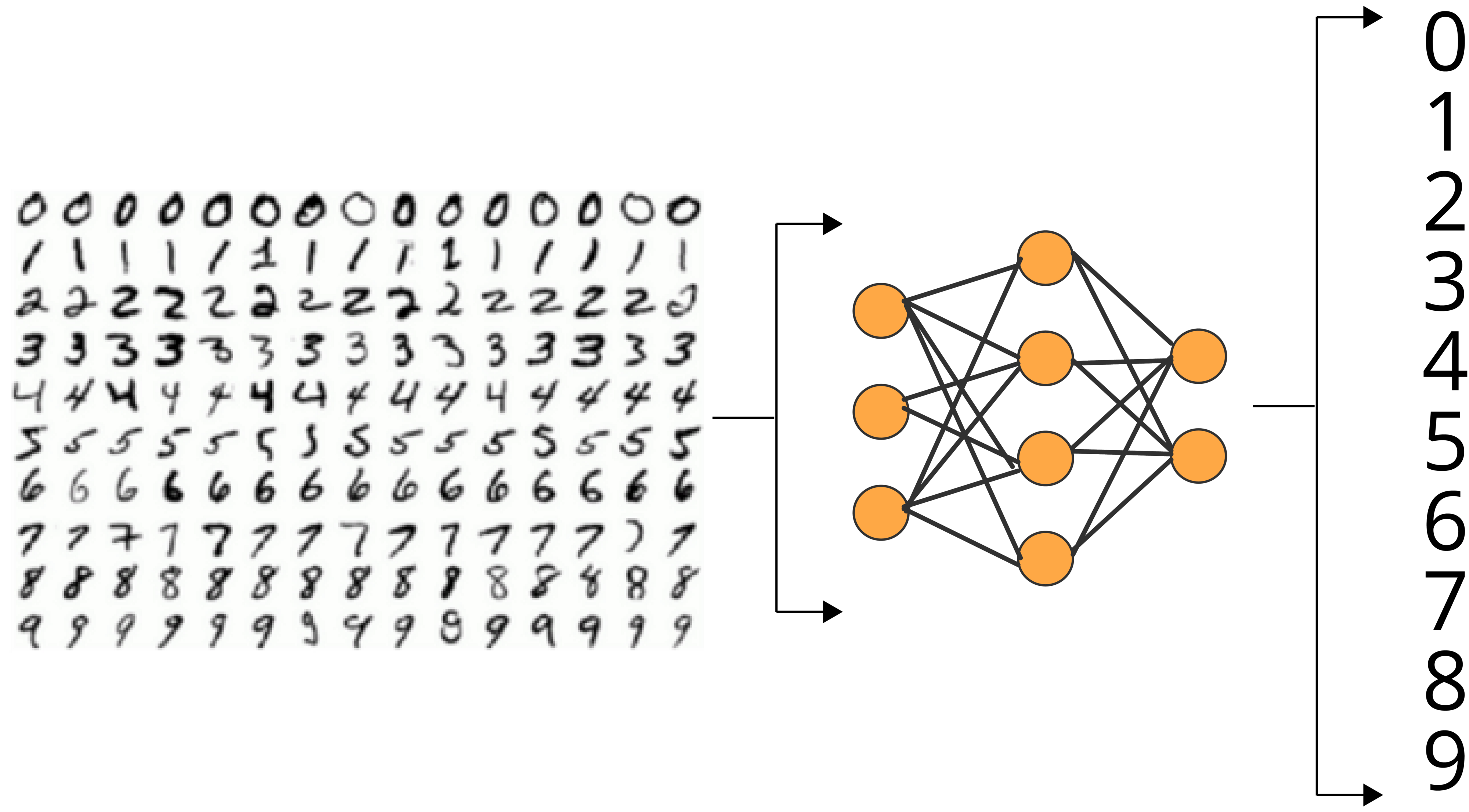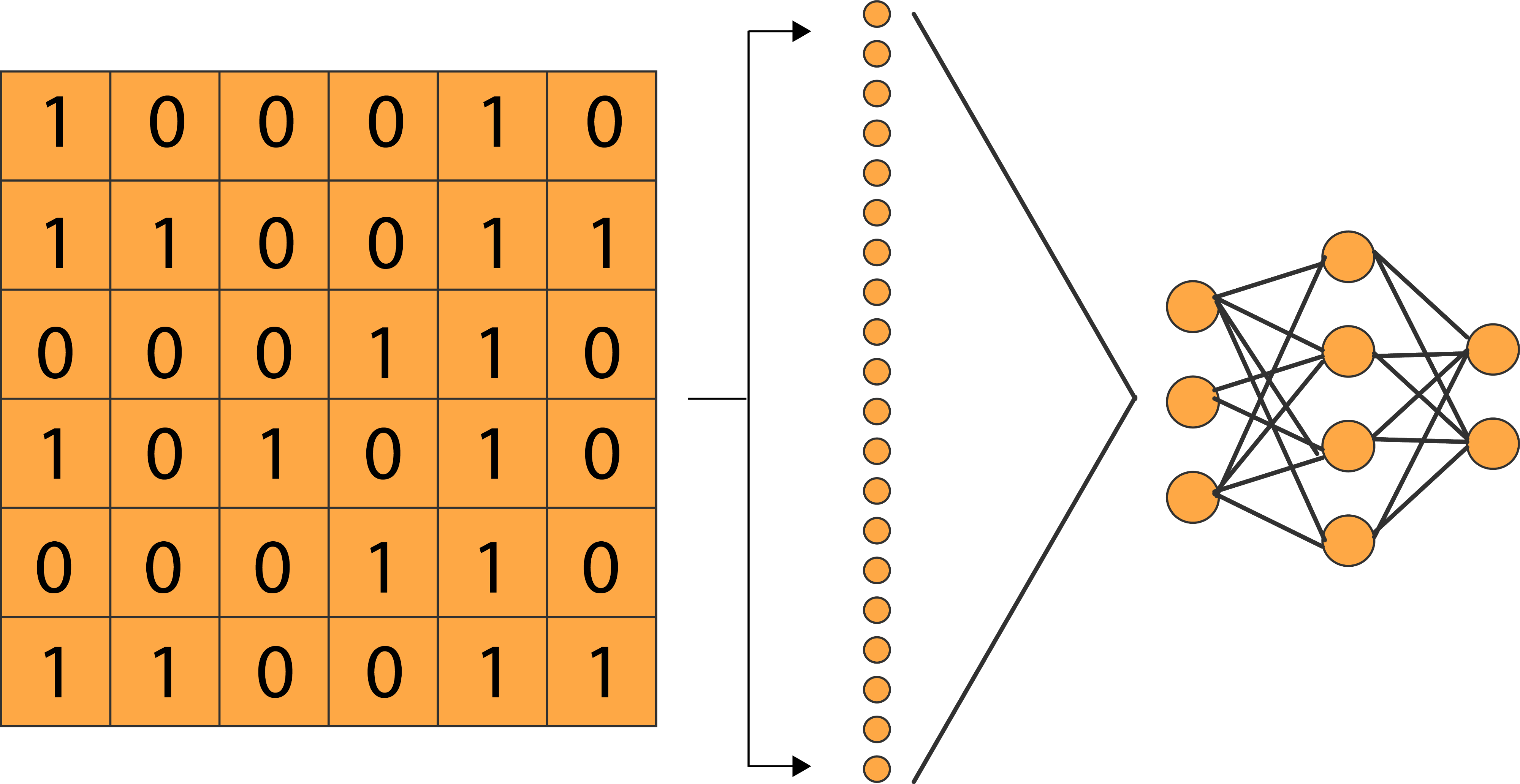# 用Keras构建神经网络的3种方法### Keras-TensorFlow的关系

#### 背景

TensorFlow发展成为一个深度学习平台并不是一夜之间发生的。最初，TensorFlow将自己推销为一个符号数学库，用于跨一系列任务的数据流编程。因此，TensorFlow最初提供的主张并不是一个纯粹的机器学习库。目标是创建一个高效的数学库，以便在这种高效结构上构建的自定义机器学习算法能够在短时间内以高精度进行训练。

#### Estimator API和Keras API• 使用Sequential API

• 使用Functional API

• 模型子类化

### 进行比较的初始代码MNIST数据集（MNIST dataset）是一个大型手写数字数据库，通常用于训练各种图像处理系统。MNIST数据库包含6万张训练图片和1万张测试图片，这些图片来自美国人口普查局员工和美国高中生。如果你想遵循完整的教程，你可以找到我的关于图像分类的单独教程：

https://towardsdatascience.com/image-classification-in-10-minutes-with-mnist-dataset-54c35b77a38d

import tensorflow as tf
from tensorflow.keras.models import Sequential
from tensorflow.keras.layers import Flatten, Dense
from tensorflow.keras import Input
from tensorflow.keras import Model
from tensorflow.estimator import DNNClassifier

(x_train, y_train), (x_test, y_test)= load_data( path="mnist.npz" )

# 确保这些值是浮点数，这样除法后就可以得到小数点
x_train = x_train.astype('float32')
x_test = x_test.astype('float32')
# 通过将RGB代码除以最大RGB值来规范化
x_train /= 255
x_test /= 255

### 构建Keras模型的3种方法

• Sequential API：当你试图使用单个输入、输出和层分支构建简单模型时，Sequential API是最好的方法。对于想快速学习的新手来说，这是一个很好的选择。

• Functional API：函数API是构建Keras模型最流行的方法。它可以完成Sequential API所能做的一切。此外，它允许多个输入、多个输出、分支和层共享。它是一种简洁易用的方法，并且仍然允许很好的定制灵活性。

• 模型子类化：模型子类化是为需要完全控制模型、层和训练过程的高级开发人员设计的。你需要创建一个定义模型的自定义类，而且你可能不需要它来执行日常任务。但是，如果你是一个有实验需求的研究人员，那么模型子类化可能是最好的选择，因为它会给你所有你需要的灵活性。#### Sequential API

model = Sequential([
Flatten(input_shape=(28, 28)),
Dense(256,'relu'),
Dense(10, "softmax"),
])

#### Functional API

inputs = Input(shape=(28, 28))
x = Flatten()(inputs)
x = Dense(256, "relu")(x)
outputs = Dense(10, "softmax")(x)

model = Model(inputs=inputs, outputs=outputs, name="mnist_model")

#### 模型子类化

• __init__函数充当构造函数。多亏了__init__，我们可以初始化模型的属性（例如，layer）。super调用父构造函数（ tf.keras.Model中的构造函数)self用于引用实例属性。
• call function是在定义层之后定义操作的地方。

class CustomModel(tf.keras.Model):

def __init__(self, **kwargs):
super(CustomModel, self).__init__(**kwargs)
self.layer_1 = Flatten()
self.layer_2 = Dense(256, "relu")
self.layer_3 = Dense(10, "softmax")

def call(self, inputs):
x = self.layer_1(inputs)
x = self.layer_2(x)
x = self.layer_3(x)
return x

model = CustomModel(name='mnist_model')

### 结尾代码

model.compile(optimizer='adam',
loss='sparse_categorical_crossentropy',
metrics=['accuracy'])

model.fit(x=x_train,y=y_train, epochs=10)

model.evaluate(x_test, y_test)

MethodSequential APIFunctional APIModel Subclassing
Loss0.087460383772850040.081315949559211730.0781003013253212
Accuracy97.82%98.06%98.20%#### 最终评估

FeatureSequential APIFunctional APIModel Subclassing
CustomizationLowMediumHigh
Difficulty to BuildEasyMediumDifficult
Layer SharingNoYesYes
Multiple BranchNoYesYes
Multiple InputNoYesYes
Multiple OutputNoYesYes
Best Suited ForBeginnersProfessionalsResarchers

sklearn机器学习中文官方文档： http://sklearn123.com/07-081638
08-211825
01-285973
10-297090
08-022183
10-122159
02-142080
09-031469
04-171825
12-181万+
04-05597
07-284662
03-1638
01-14189
04-10466
09-021194